DataFiller help

This tool serves as a wizard to make easier to introduce the data to the optimization application.  The main elements of the application are shown below: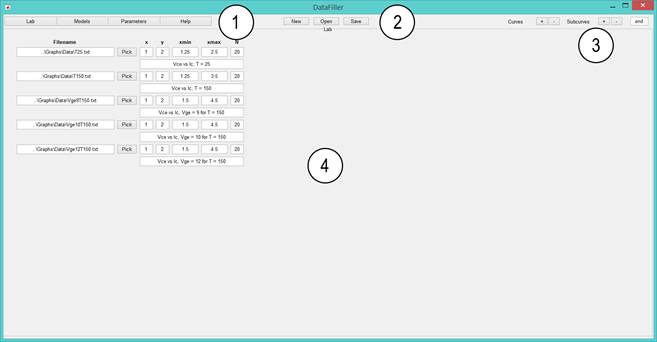1 You can change the section here or access help. 2 You can create a new file, open or save. You can use this tool as a way to save data to the Optimization Application but also as a way to read it in an easier format. 3 You can add and remove elements to the list using the +- buttons. The curves will add a new curve, that is a new entry containing each own file. If you want to add an entry within the same file you can add a subcurve instead. For example if a file contains more than one curve you can then add subcurves within the same file to put all the variables. If you want to add/remove elements in an entry other than the last simply put the curve number in the text field to the right. 4 The content for each tab is presented here and is explained in the following pages.

# Lab

You can add curves by using the +- buttons on the top right corner: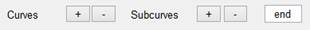Press the + button in curves to add a curve. The application will look like this:The first parameter is the file containing the data. You can type the location or use the pick button. If you want to create a new curve from data from a datasheet for example you can do it with Data Picker. Next is the position of the x-variable and y-variable. The file with the data must be structured in a .txt with columns where each one represents one variable. Therefore you have to put in xvar and yvar the index (starting in one) of the variables corresponding to x and y axis for the curve you add following the same order as in the file specified. You continue by setting the x-axis limits in xmin and xmax and the number of points to use in N. Finally you can give the curve a name. If the same filename contains more variables you want to use you don’t need to add it again as a separate entry. You can simply add a new subcurve where you will specify the last 6 parameters again.

You can remove curves and subcurves at any moment by using the – buttons. You can select a curve by typing its number on the text field to the right of subcurves on the toolbar. That way you can place curves after the one you choose or remove that particular one.

# Models

Press the model tabs to go to models. For help relating how to create models refer to this. Then you can add curves and subcurves the same way as you did in Lab. Because some of the parameters are already registered, you only need to specify the x and y-axis index. The screen will look like this: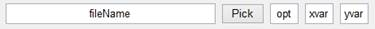Select the filename and put the axes. The xvar represents the index of the data produced by .print in the .cir file and same for the yvar with the y-axis. You can again add more subcurves if one model has more than 1 set of points.

The opt parameter is optional. Leave it blank or set to ‘opt’ so the application ignores it. This parameter controls extra options for the models. Currently the options allowed are <i>, <M> and <s> which do the following.

·         <i> is used to assign importance to the curves of a model. By default all the curves have the same weight arbitrary chosen as 1 if this option is not used. That is, when the average global error is computed by adding the curves each one has the same weight independent of the number of points used or segment size. If you want to specify a different importance use this function. You specify the importance by placing each importance for each subcurve of each model you want as a vector. For example, imagine you want to say that each individual AC test is worth twice as much as each individual DC. Then you would put <i=2,2> in the AC models as the rest of the models have the default weight of 1. Then when the objective function is created the result would be: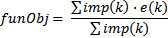This means the objective function is now an average weighted error.

·         <M>: It will add a shift between the first variable of the model so that it can shift that variable. It will shift it in such a way that the maximums of the model second variable (that is the first y-variable) and the experimental will coincide. It can be illustrated as shown in the following image: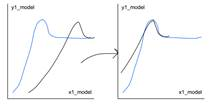·         <s>: The curves from the model will be compared with the lab curves but shifted with an optimum shift so the error is the minimum in the model first curve. This can be illustrated as: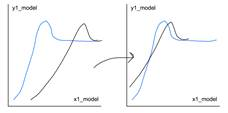# Parameters

In this tab you can specify the parameters by using the +- buttons on the top right corner. When you add a new parameter it will look like this: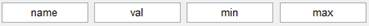Type a name for the parameter starting with a letter and then it may only contain letters, numbers or underscores. If you expect the parameter to vary in orders of magnitude it can be a good idea to make a logarithmic transformation. To do so simply finish the parameter name with _log. The val controls the initial parameter to use and min and max the limits. You can remove any parameter by typing its position in the textfield to the right of the +- buttons and then pressing the – button. Additionally you can add a parameter after another by selecting the parameter before using the same text field and pressing +.

# More

In the last tab you can specify the constraints as well as the optimization method to use.

## Constraints

You place each constraint separated by a new line. You can leave it blank if there aren’t any. Apart from the lower and upper bound constraints from the parameter tab you can specify linear constraints like: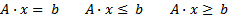You can type them using the same names you used in the parameter tabs or by placing the symbol # followed by the number the variable has as a position starting on one. For example, let’s imagine we have the following set of parameters:

{t2,tb,t3,t4,kpm,vtom,rsm,gammab,gamma3,gamma2,gamma, gamma4}

If we want to put a constraint such as that the sum of the gammas is equal to one you would type:

gammab+gamma+gamma2+gamma3+gamma4=1

The order of the variables does not matter and you can also multiply or divide the variables by constants.  Inequality constraints are written the same way by simply replacing the equal symbol, =, with either <= or >=.

## Optimization method

You can choose between 3 different optimization methods that are briefly described below. You can get more information on the documentation of Matlab.

1.       Fmincon: It’s a local gradient-based method. This means the system will be approximated with a model using the gradients of the objective function with respect to the parameters. This approximations typically work better in linear problems but can be applied on non-linear problems as long as the functions are smooth enough. The advantage is that they’re one of the fastest methods available with the disadvantage that the optimum found (the one that minimizes the error between model and experimental) may not be the global minimum and the smoothness of the objective function used. That means the parameters you find may not be close to the real ones but in most cases the results are good as long as the initial point is “close enough”. It is recommended to start with this method and if it doesn’t work continue with others. The results for this methods may depend on the initial parameters so if the parameters vary a lot this method may not work or you may use it after you have a general idea of where the parameters may be. This method may converge in a few hours.

2.       Global Search: It’s a gradient-based method but it is global. The method will basically start at different starting points so different minimums may be found. As it is a gradient based method it will work well in smooth problems but if the functions change a lot suddenly it may not work. It’s an heuristic and the probability of finding the global minimum increases with the number of starting points and stage points selected. However, increasing those numbers will also require more iterations so a compromise may be used. This method may converge in a day.

3.       Genetic algorithm: This is the most generic method available. It is also the slowest. It is a heuristic method that mimes evolution. A lot of random different starting points are chosen and the method will combine them together trying to find the most “fit ones”. There are different generations and different set of parameters are combined to have “children” that share characteristics of the parent (that is the parameters found are in between the parents). It also induces mutations (random modifications on the children) to try to find every possible combination. If it is well designed it will find the global minimum even with functions that are not smooth such as discrete or functions that may not be defined at some points. It is however much slower and it is only recommended as the last available option. This method may converge in days or even weeks.

Apart from the method the options that can be set to the different algorithms are hidden to the user for convenience. The options chosen may be adequate for a general problem but they can always be modified by changing them through the code using the optimoptions for the first two and gaoptimset for the genetic one in MainApp.m.

The problem has been scaled as follows:

·         The parameters are divided by the initial value. That means the algorithm will see [1,1,…] as initial parameters and all the parameters will “be similar” which is always recommended. In the case of a logarithmic transformation being made, however each log-variable will be transformed into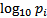. This means the variable will have a value as seen by the algorithm close to the closest 10-power which again is close to one and thus is also scaled. What this does is that when the variables grow all the estimations will take bigger differences while when they’re smaller they are reduced contrary to linear parameters that always take the same differences. It also makes sense because if a variable has a big value you would expect a degree of certainty close to that value but not much bigger or smaller. When the variables do not change that much the linear transformation is enough, but for variables that can change a lot in orders of magnitude this is preferred.

·         The objective function will typically be less than one and is directly an average error. It is calculated as the difference between experimental and model and scaled by the experimental value at that point. It can be easily seen that if a point is close to zero the error will be very big. To prevent high error amplifications in values close to zero a small modification is therefore done. The value to be used in the scaling cannot be less than a certain percentage of the mean of absolute values of the whole experiment. The whole implementation can be read in getErrorNi.m.

Under this conditions the default values chosen by Matlab for the algorithms seems appropriate except the finite differential used on the estimation of the gradients. Because the data always have noise it is not a good idea to use a value as small as the proposed of 1e-6 and a value of 2-5% seems more appropriate. The measurements may also be filtered before giving them to the tool but this is not usually needed.